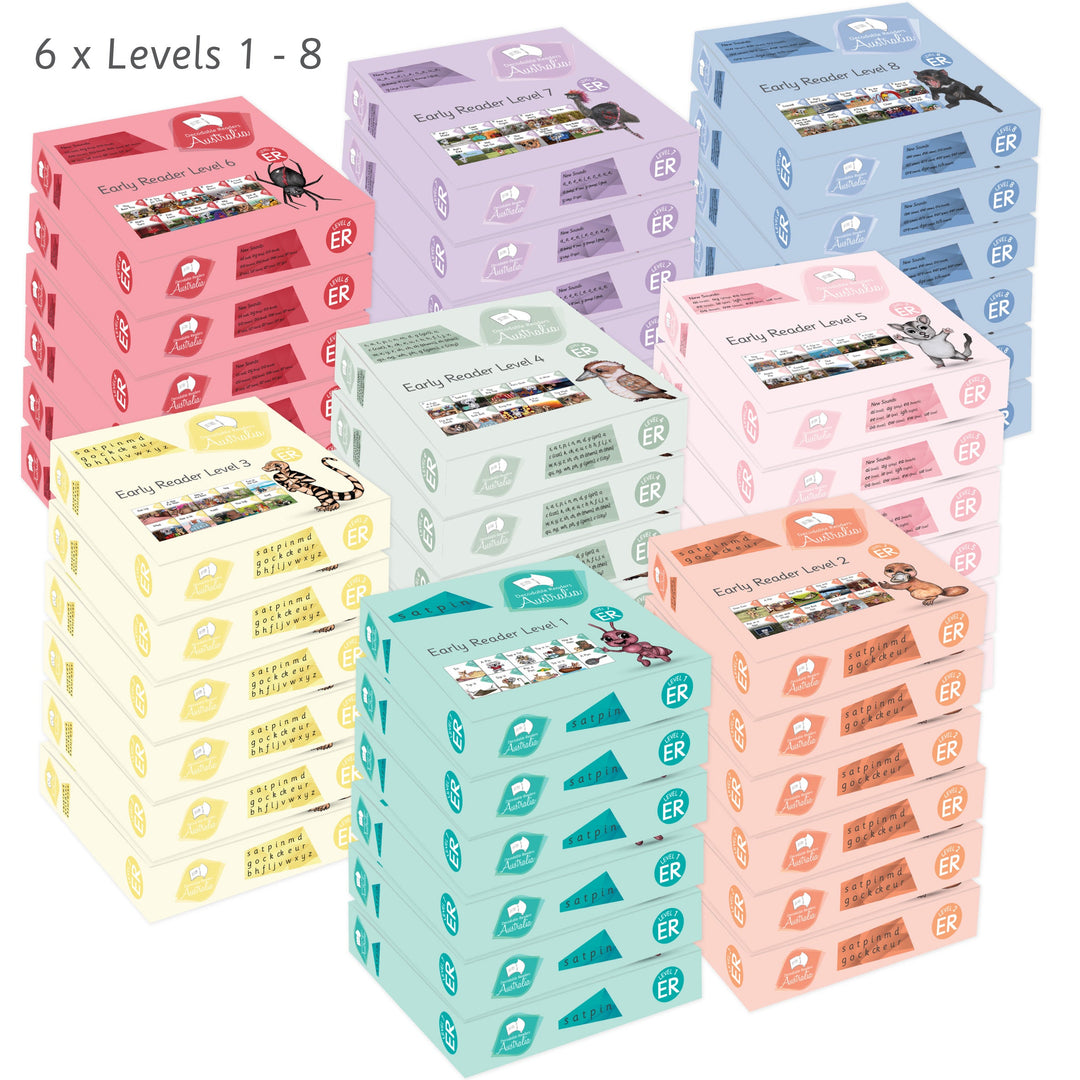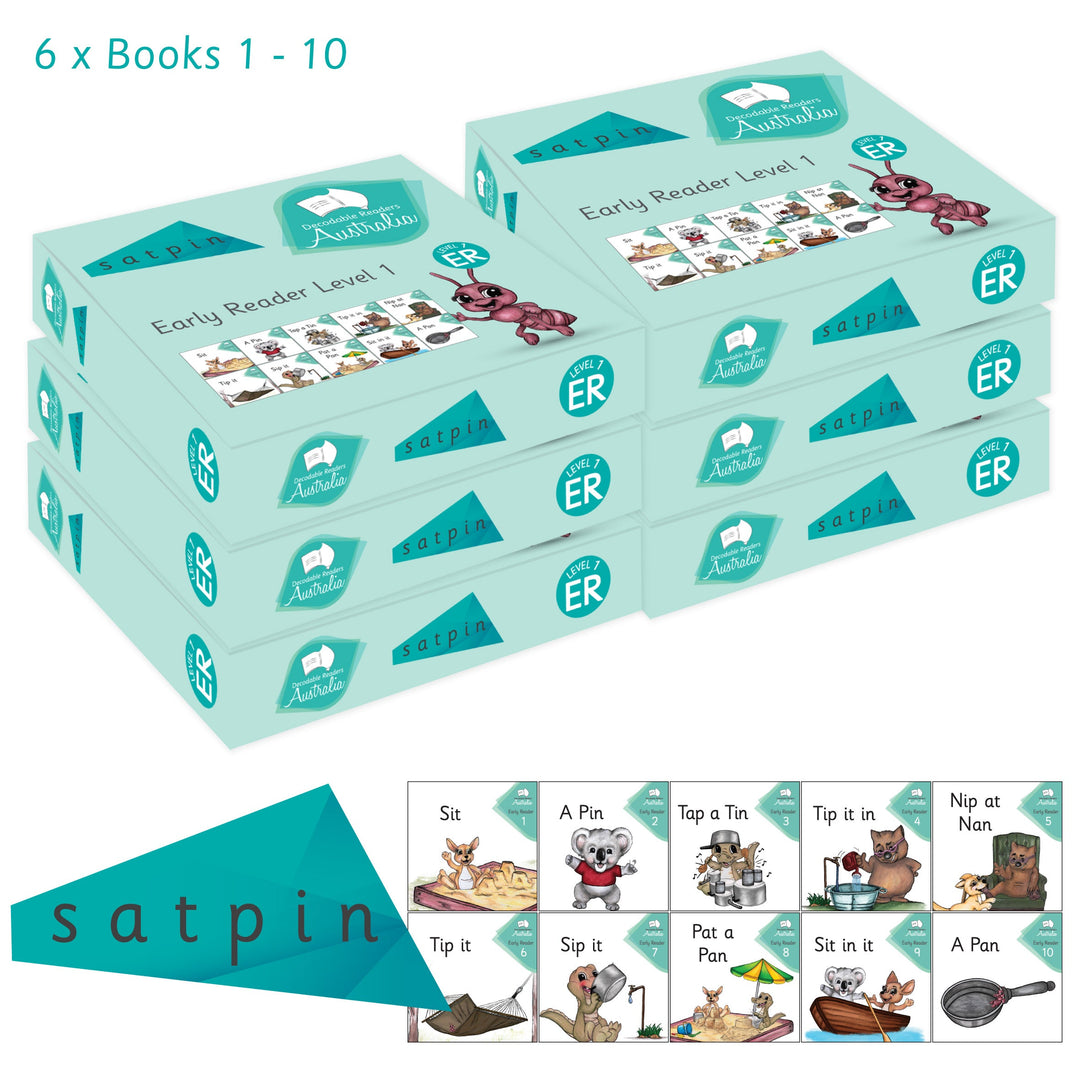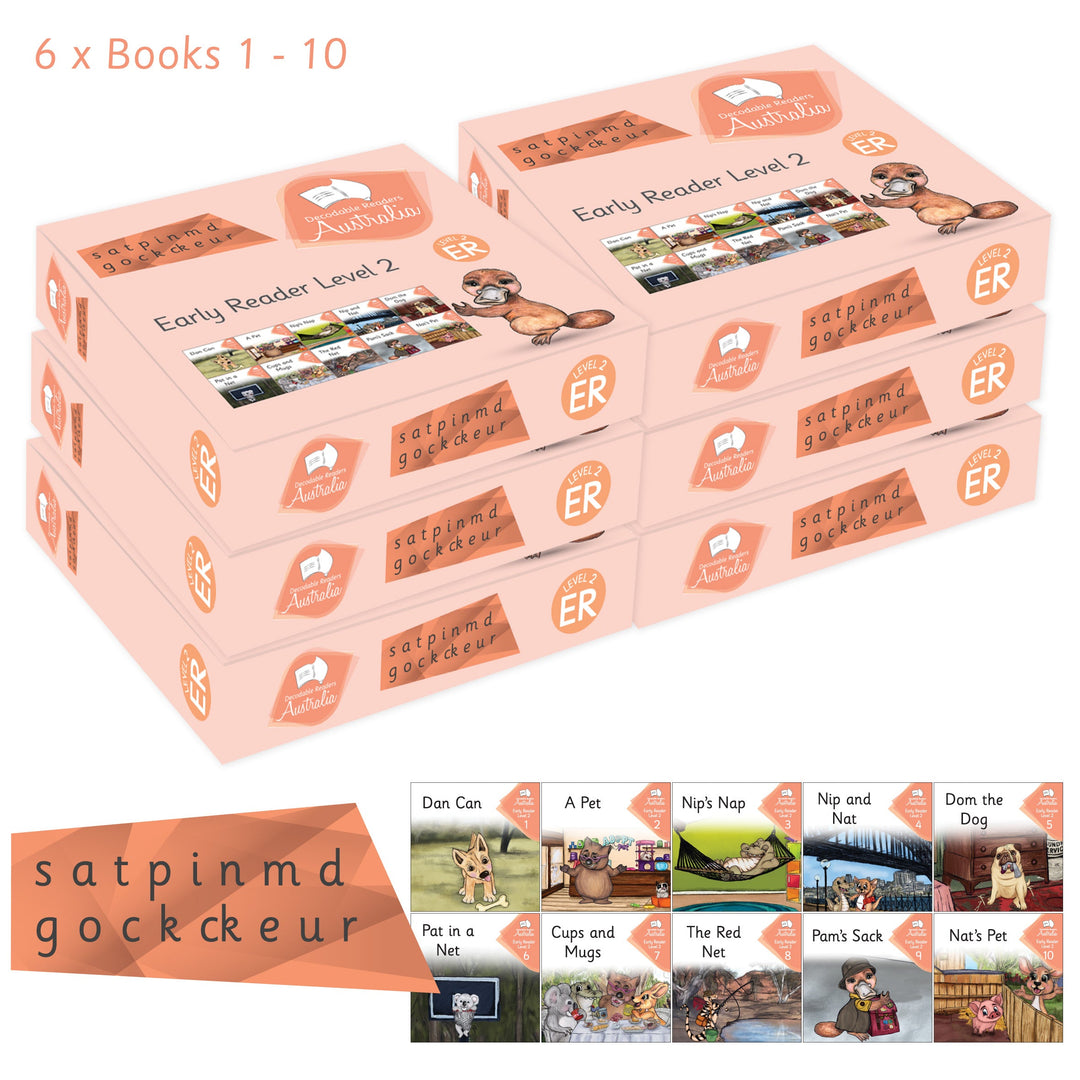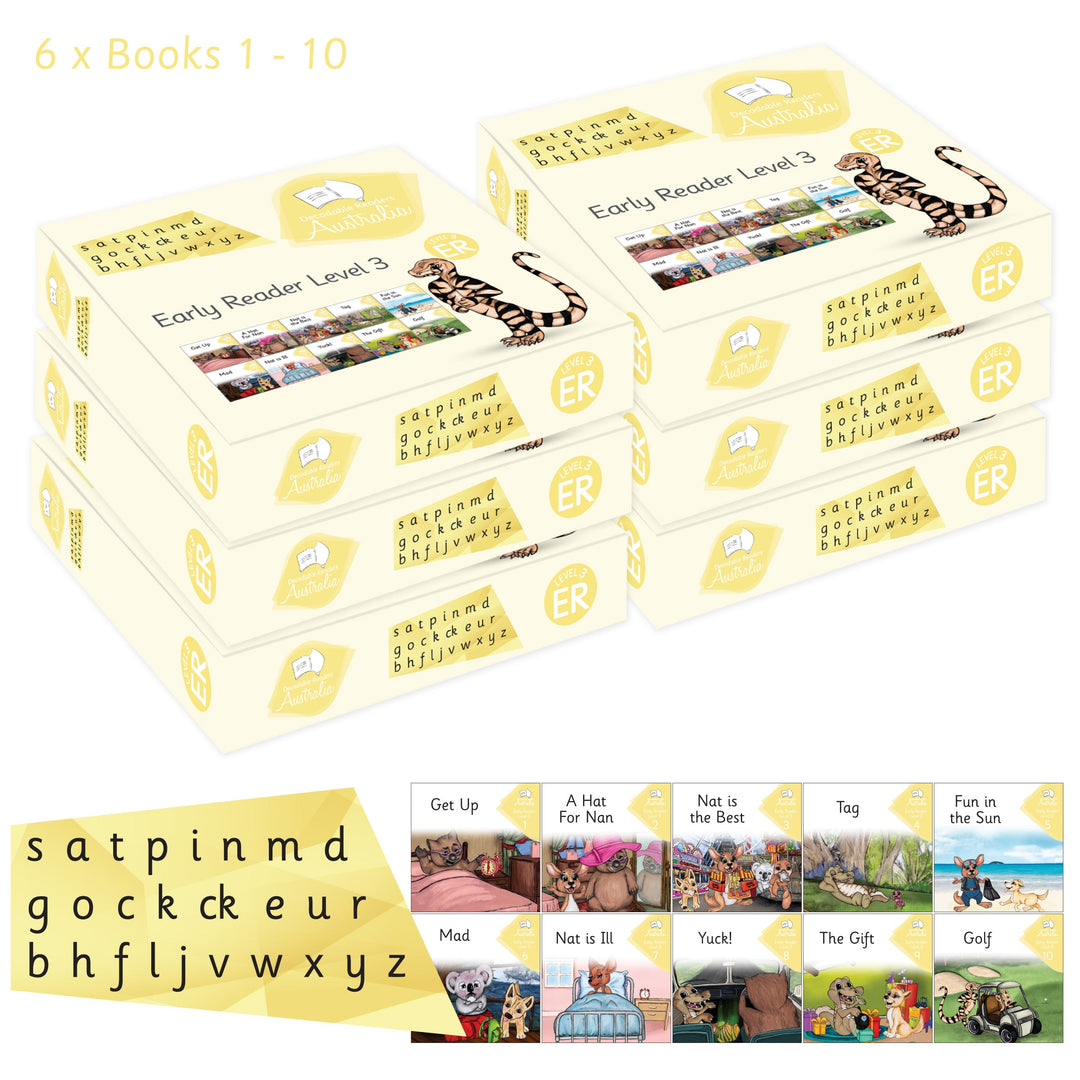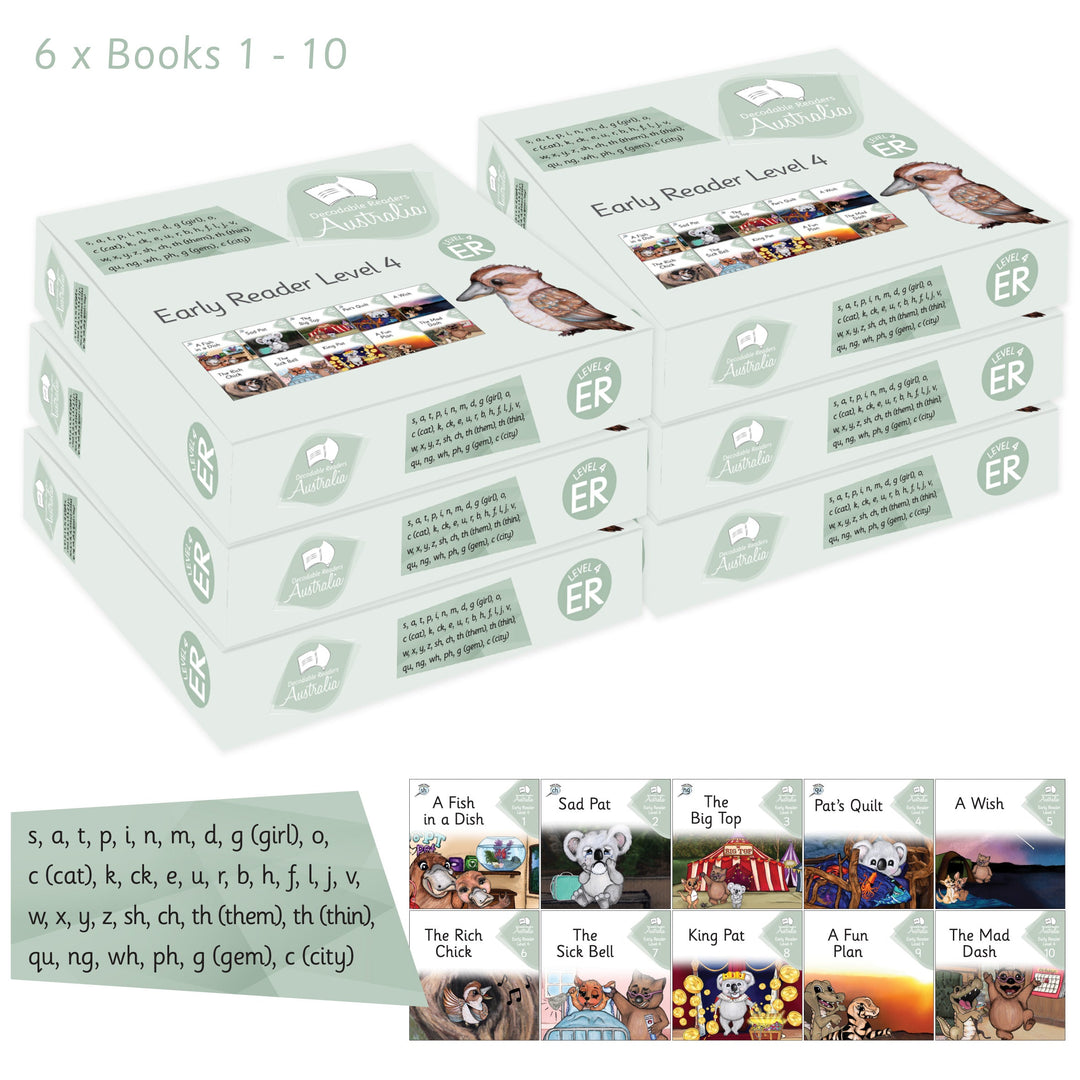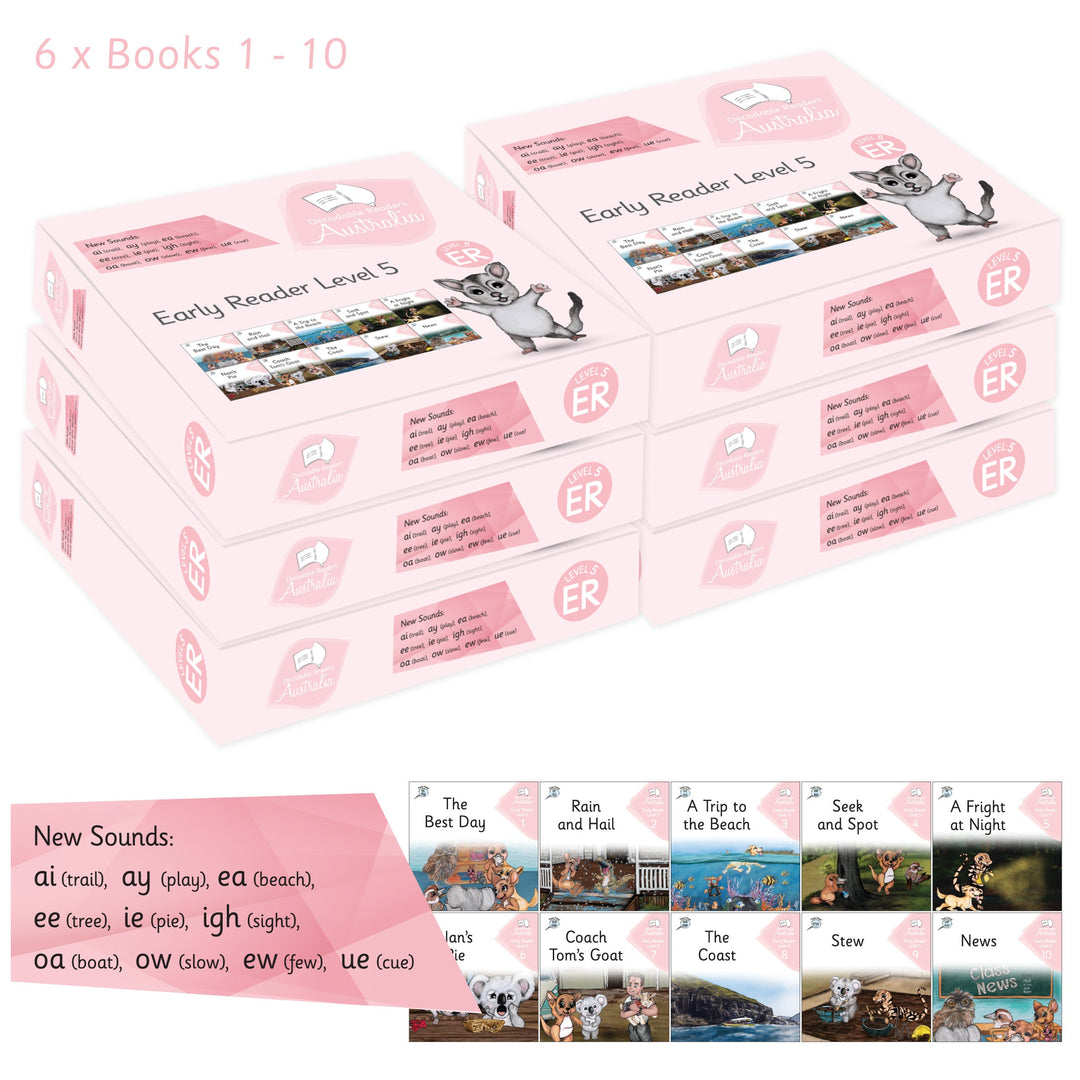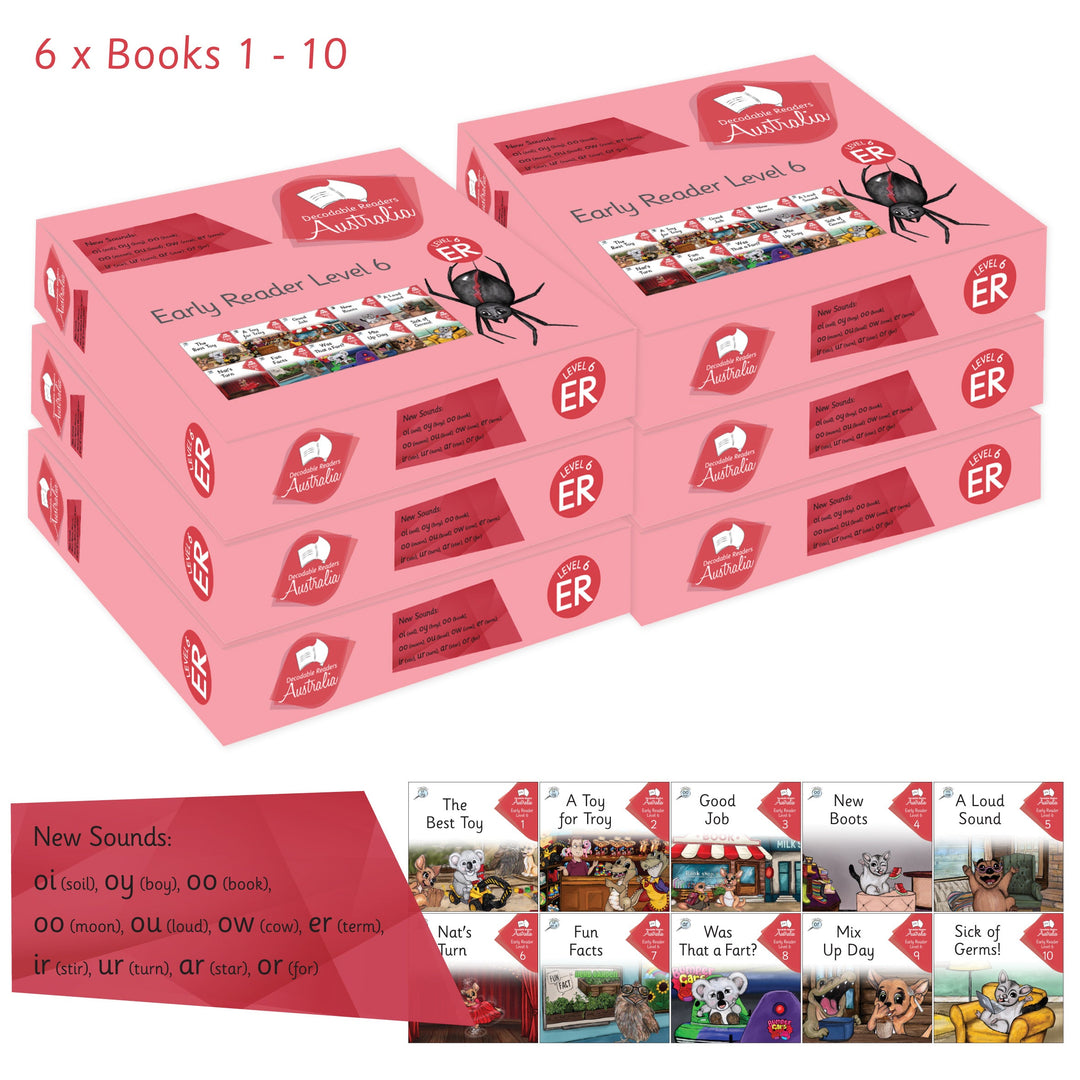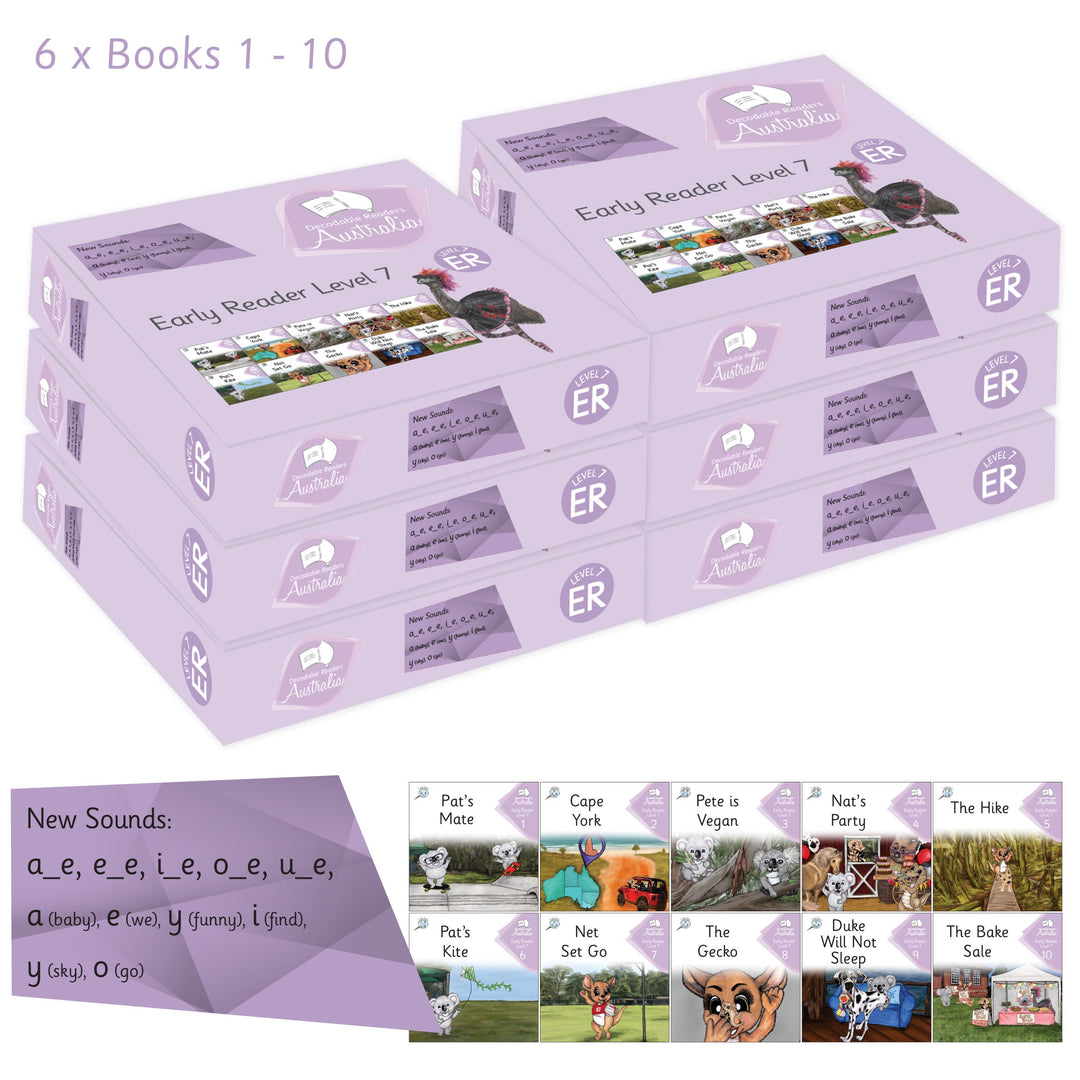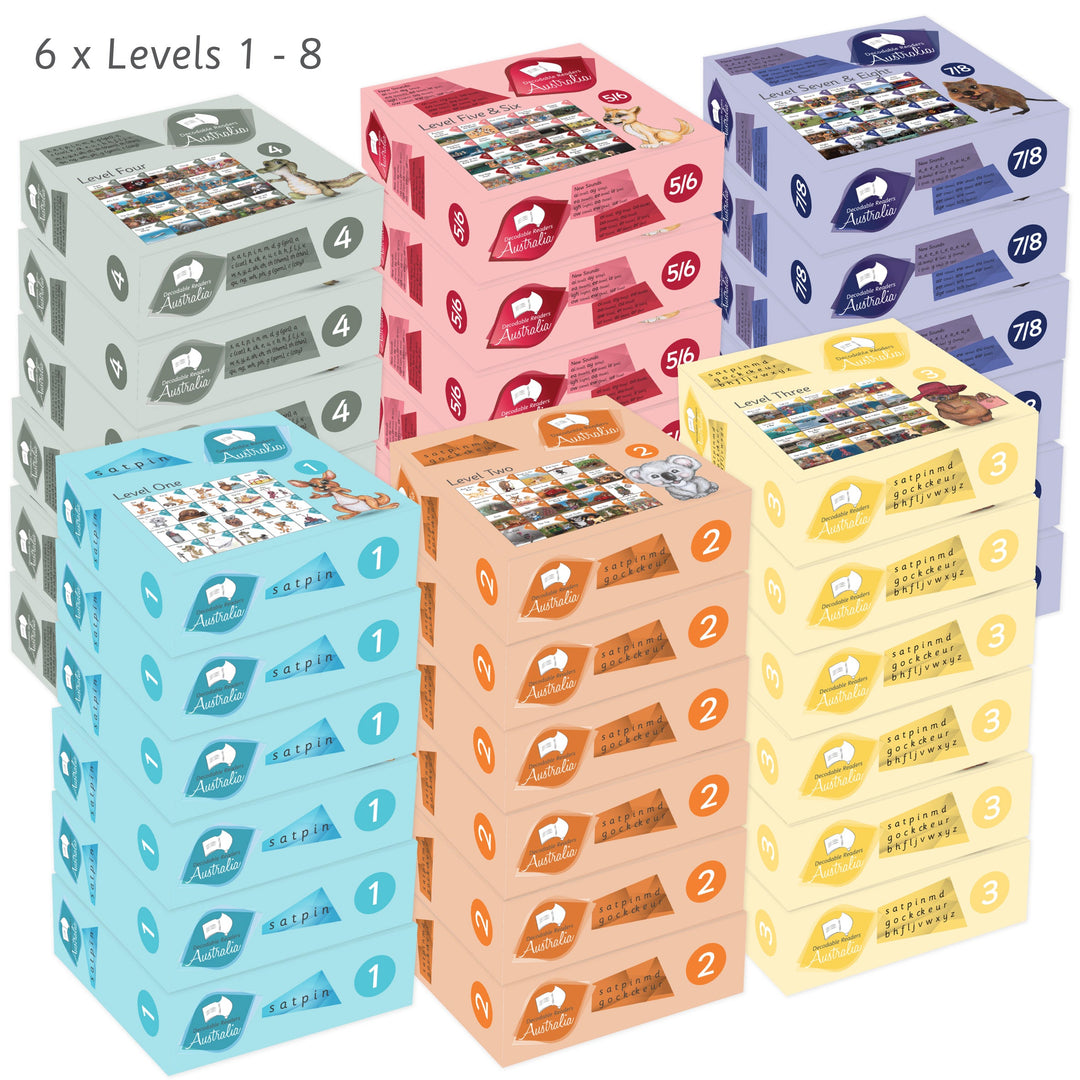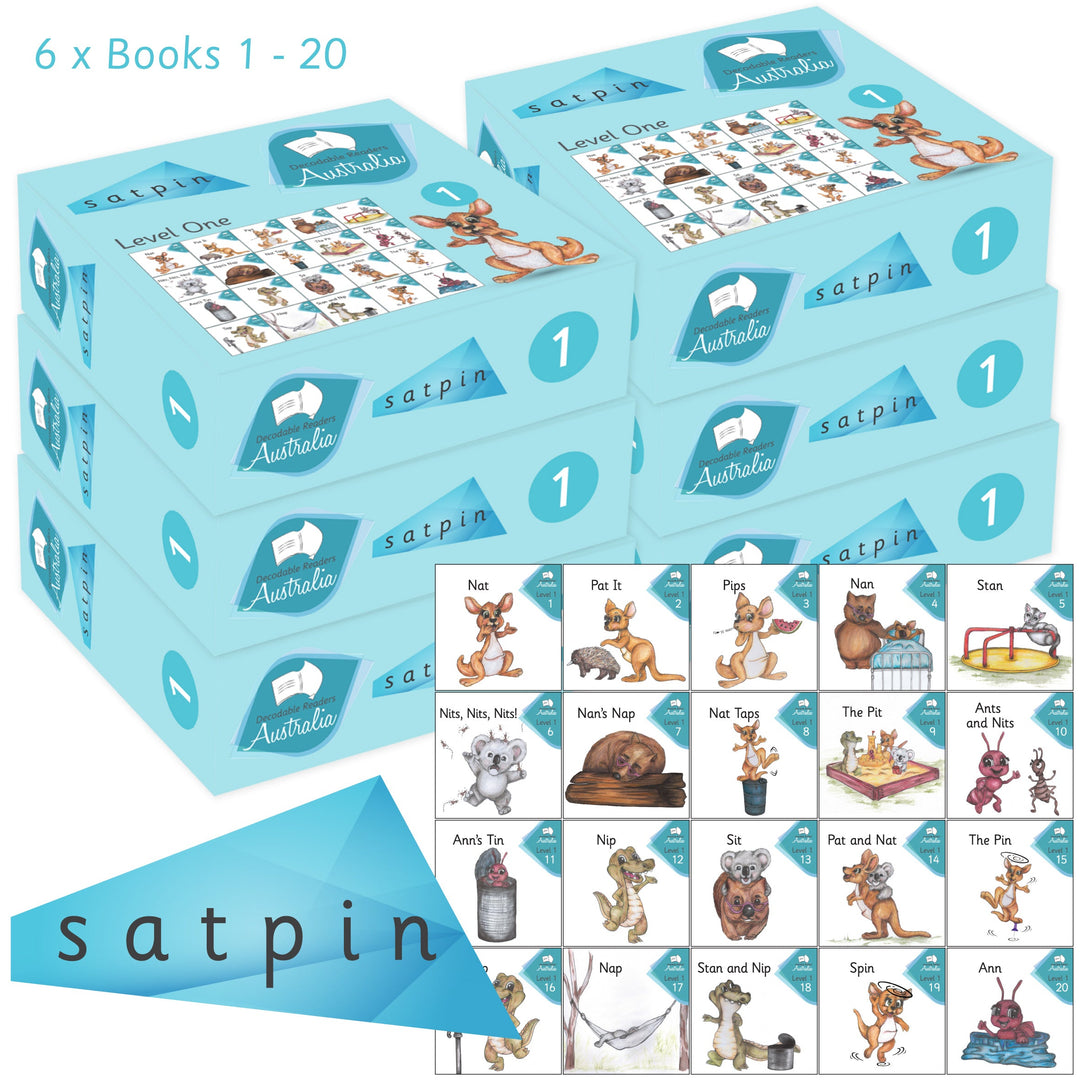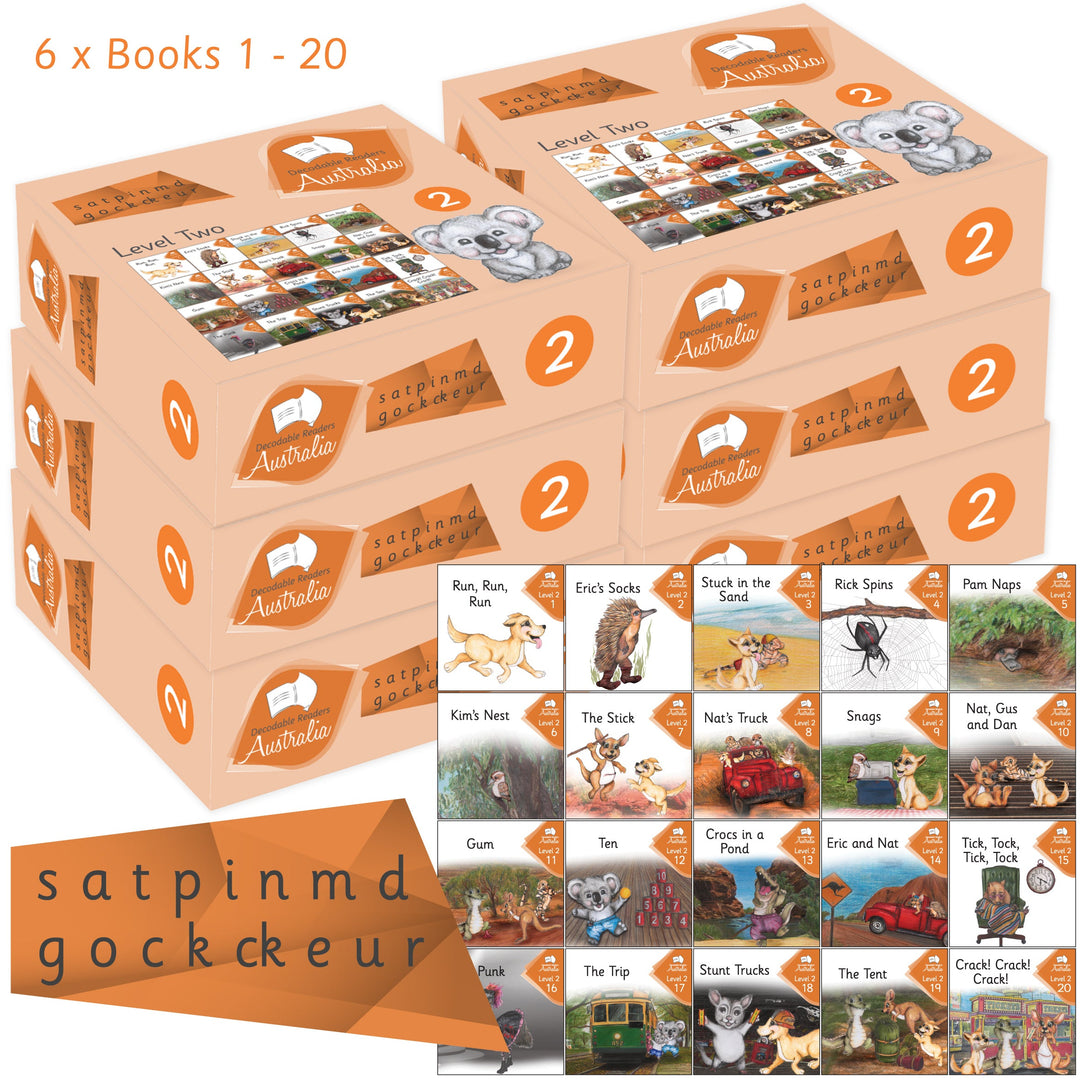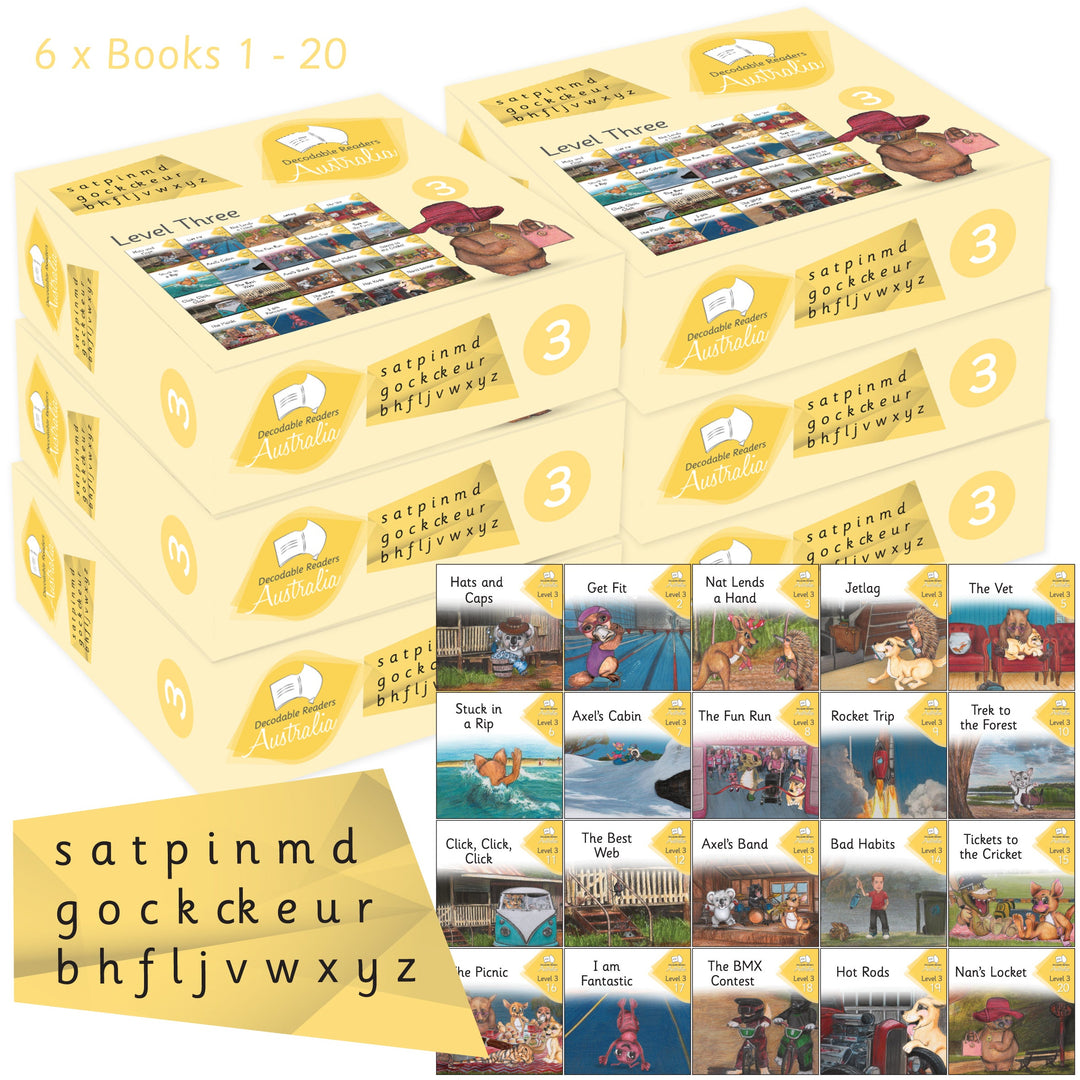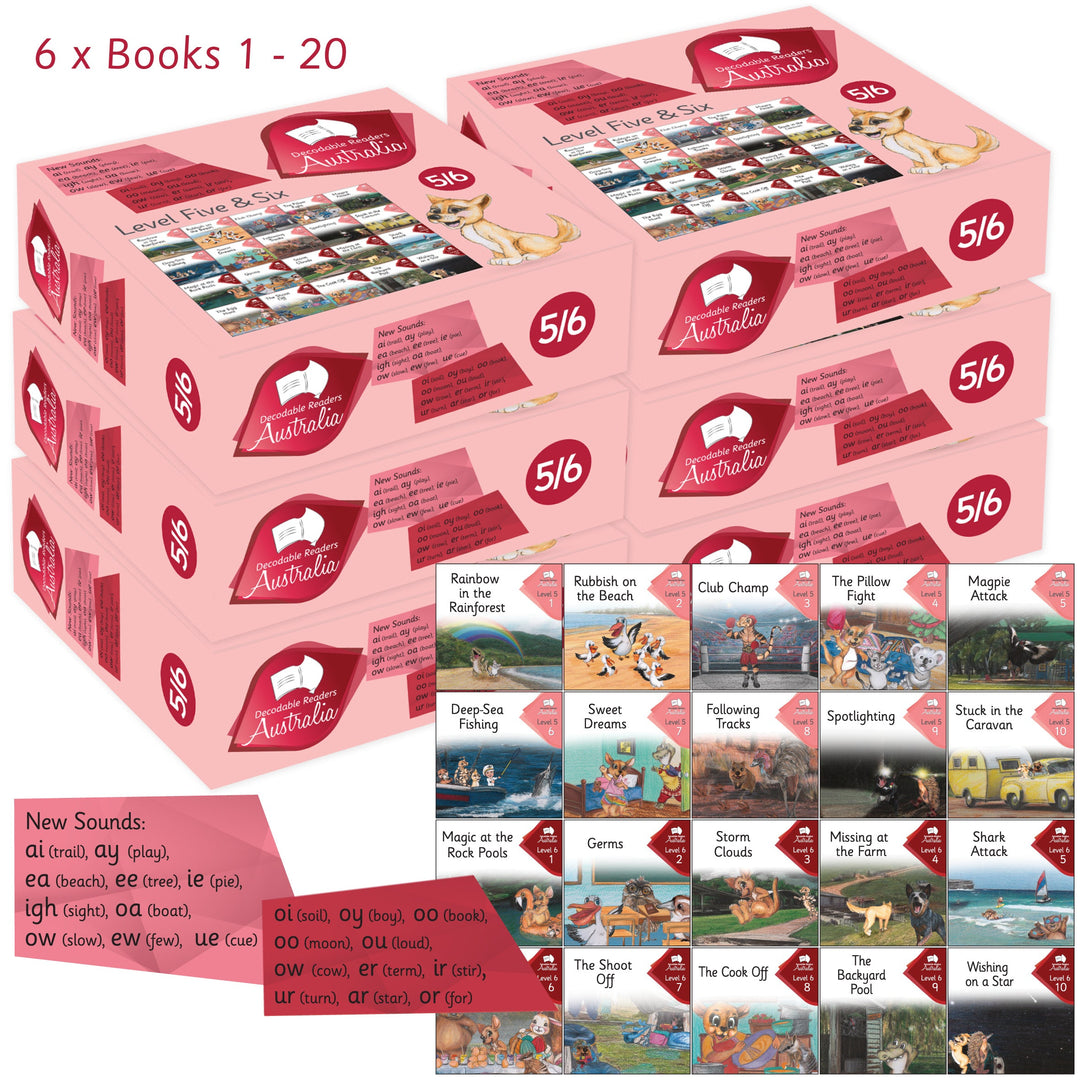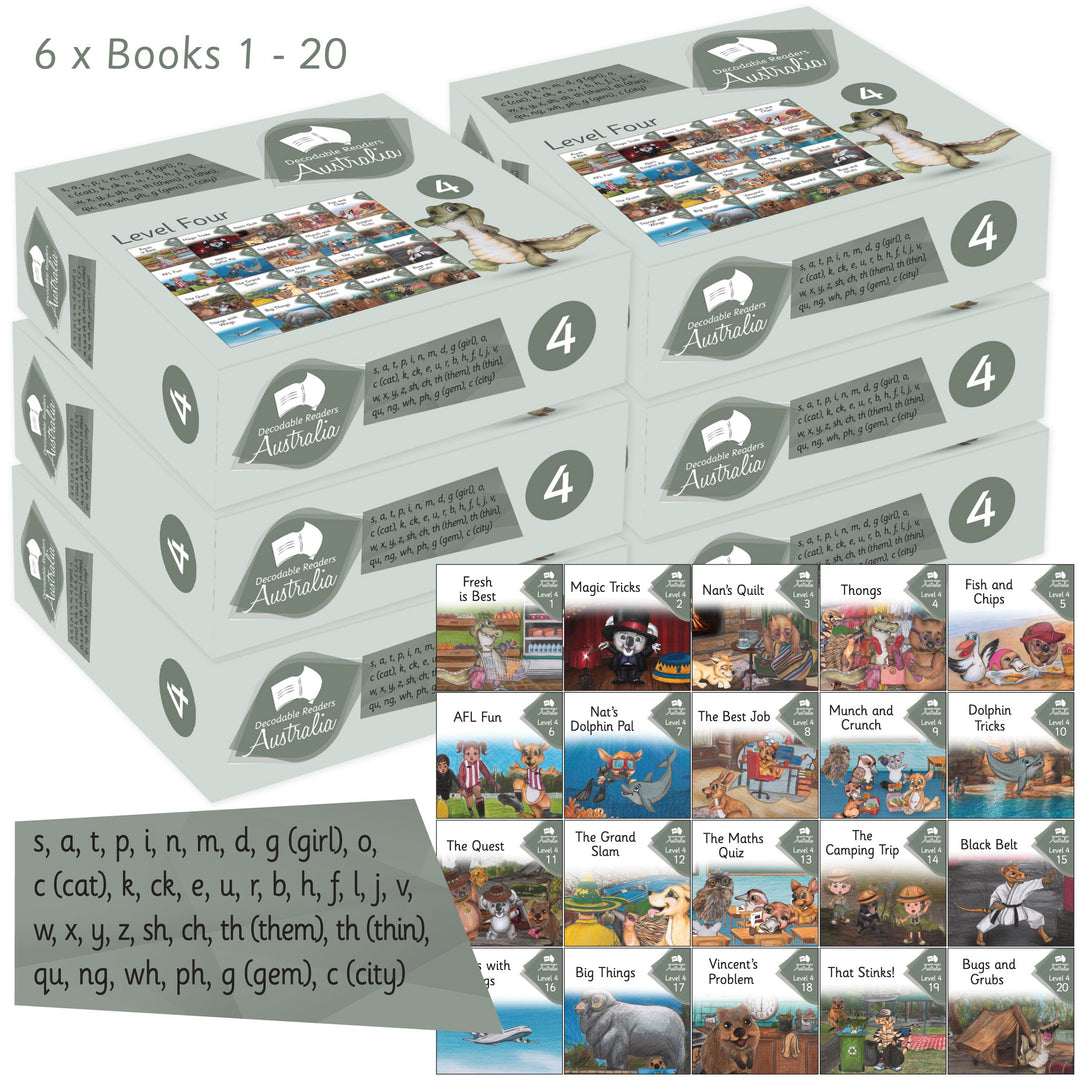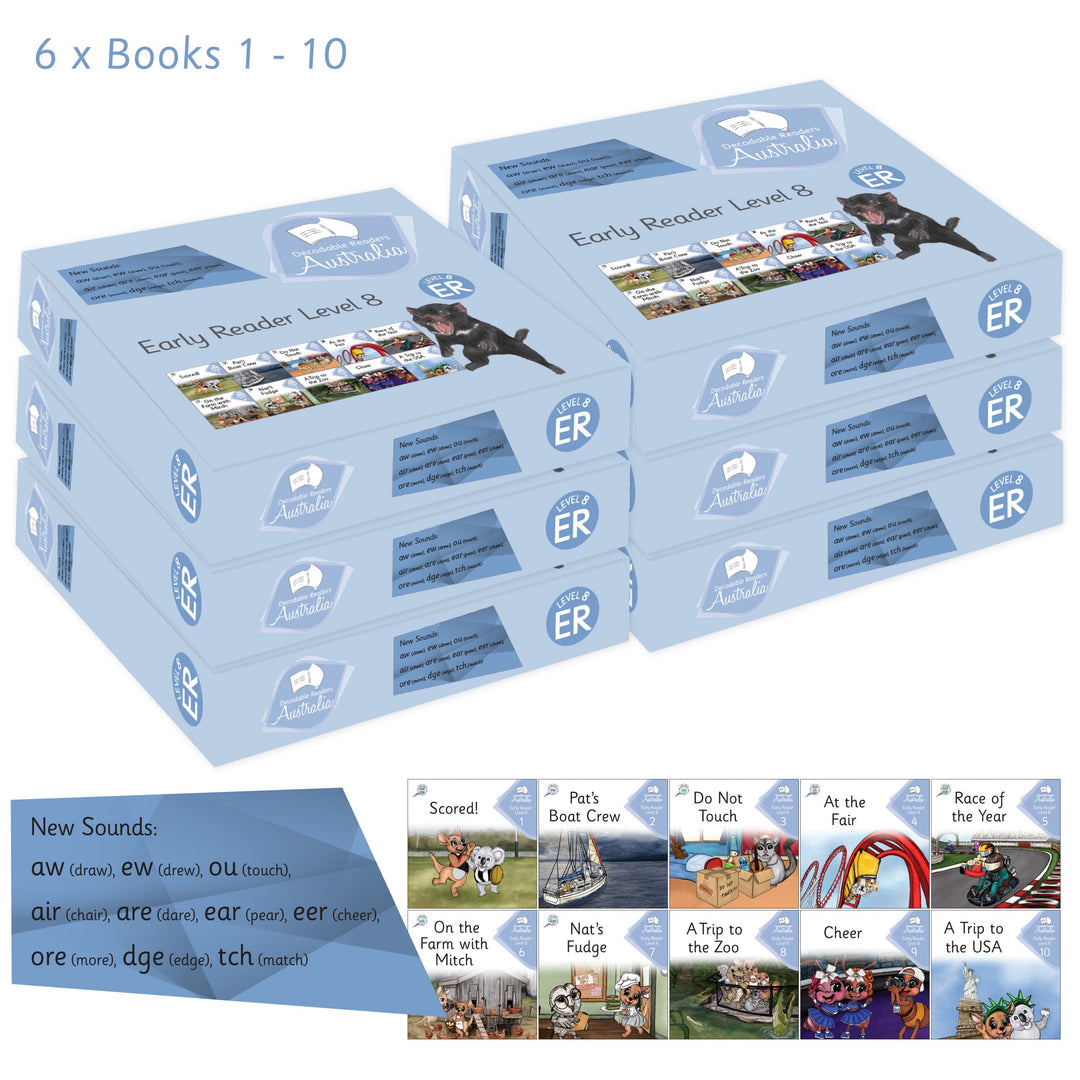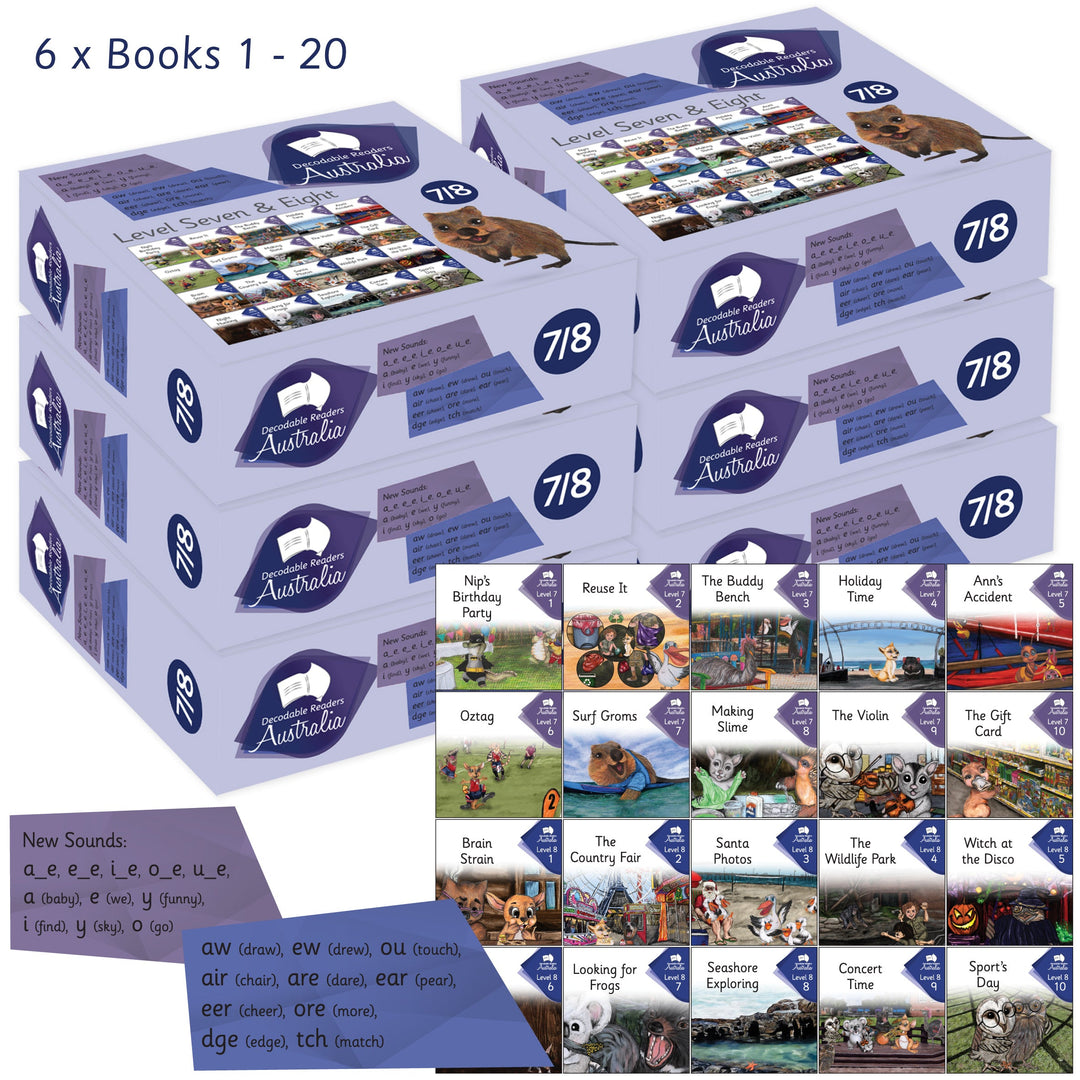# Combined Classroom Complete Set - Early Readers + Main Fiction Series - Guided Reading (6 sets of each level)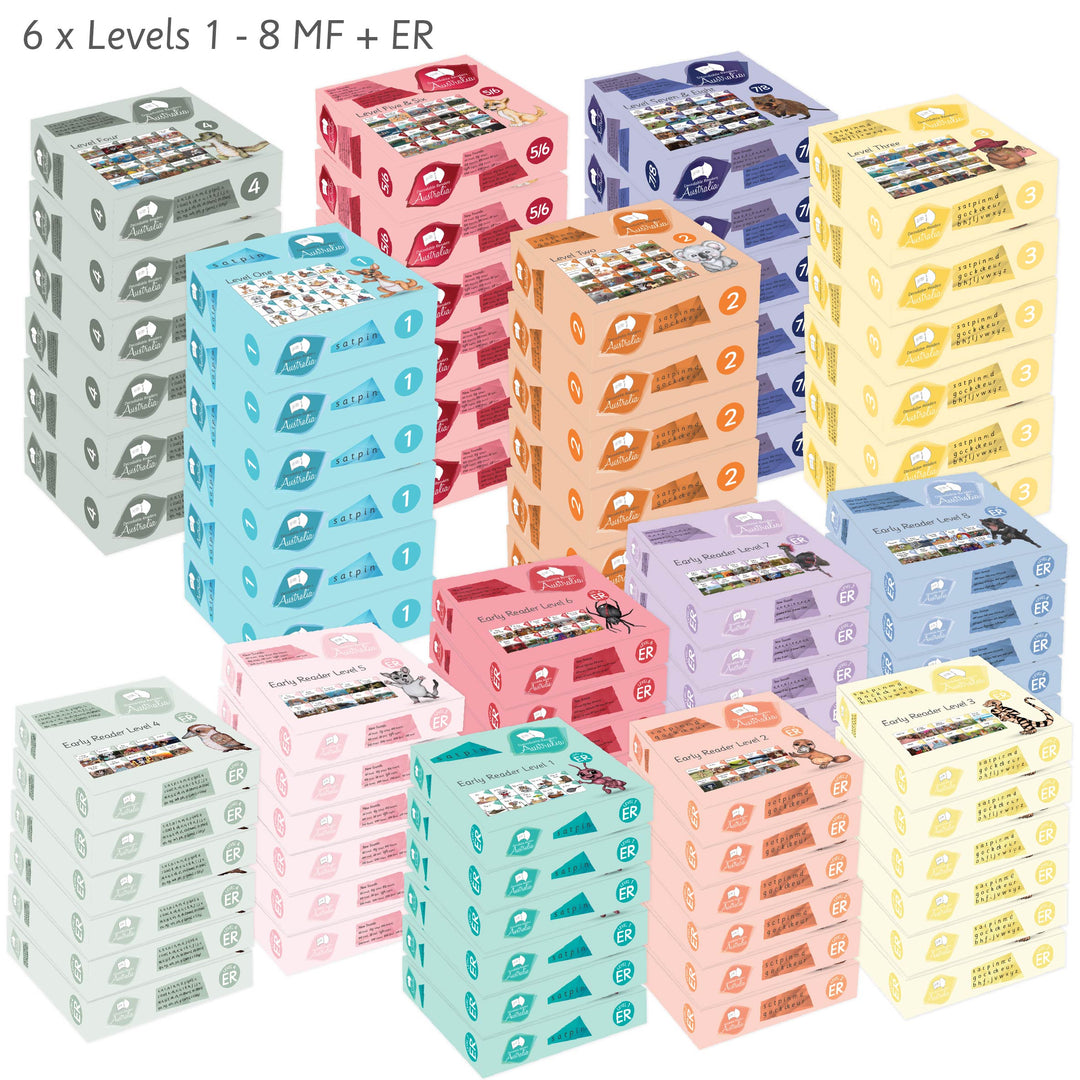\$6,400.00 AUD

\$6,900.00 AUD

Combined Classroom Complete Set - Guided Reading
Levels 1 to 8

Resource your Early Years Classrooms, Learning Support and Library!

6 x Complete Individual Early Reader Fiction Series Sets =
6x Level 1 = 60 Books
6x Level 2 = 60 Books
6x Level 3 = 60 Books
6x Level 4 = 60 Books
6x Level 5 = 60 Books
6x Level 6 = 60 Books
6x Level 7 = 60 Books
6x Level 8 = 60 Books

= 480 Books

6 x Complete Individual Main Fiction Series Sets =
6x Level 1 = 120 Books
6x Level 2 = 120 Books
6x Level 3 = 120 Books
6x Level 4 = 120 Books
6x Level 5/6 = 120 Books
6x Level 7/8 = 120 Books
= 720 Books